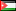##### Application of Genetic Algorithm for Synthesis of Large Reversible Circuits using Covered Set Partitions
Publication Type
Original research
Authors

We present the results of application of Evolutionary Algorithms to the problem of synthesizing quantum circuits which belong to the class of reversible circuits, represented as an input/output mapping vectors. The paper specifically focuses on large quantum circuits where many valid solutions exist in an exponentially inflating search space. Valid solutions represent the set of all input vector permutations (arrangements) which satisfy the circuit specification. The search space for circuits with large number of variables grows exponentially making it impossible to discover the set of optimal solutions. The paper compares three methods for selecting valid solutions of input vector sequences: 1) randomly, 2) genetic algorithm, 3) Tabu search. The objective function calculates the number of elementary quantum gates needed to represent the solution such that lower number of gates represents better solutions. In addition to the choice of selection algorithm, we illustrate the impact of using different partition depths for the Covered Set Partitions algorithm used to construct valid input vector sequences.

Journal
Title
The Fourth IEEE International Symposium on Innovation in Information Communication Technology (ISIICT 2011). Amman, Jordan. November 2011, Volume: 1
Publisher
--
Publisher Country
JordanPublication Type
Both (Printed and Online)
Volume
--
Year
2011
Pages
--